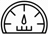# AverageThis section comprises quantitative aptitude multiple choice questions with their solutions and Explanations on average. To browse other quantitative aptitude sections, please click here

# There are 100 students in 3 sections A, B and C of a class. The average marks of all the three sections was 84. The average of B and C was 87.5 and the average marks of students in A section was :

There are 100 students in 3 sections A, B and C of a class. The average marks of all the three sections was 84. The average of B and C was 87.5 and the average marks of students in A section was : [A]20 [B]25 [C]30 [D]35 Show Answer 20 Numbers of students in section ..

# The average weight of first 11 persons among 12 persons is 95 kg. The weight of 12th person is 33 kg more than the average weight of all the 12 persons. The weight of 12 persons is :

The average weight of first 11 persons among 12 persons is 95 kg. The weight of 12th person is 33 kg more than the average weight of all the 12 persons. The weight of 12 persons is : [A]97.45 kg [B]128 kg [C]128.75 kg [D]131 kg Show Answer 131 kg Let the weight of 12th ..

# The arithmetic mean of the following numbers 1, 2, 2, 3, 3, 3, 4, 4, 4, 4, 5, 5, 5, 5, 5, 6, 6, 6, 6, 6, 6 and 7, 7, 7, 7, 7, 7, 7 is :

The arithmetic mean of the following numbers 1, 2, 2, 3, 3, 3, 4, 4, 4, 4, 5, 5, 5, 5, 5, 6, 6, 6, 6, 6, 6 and 7, 7, 7, 7, 7, 7, 7 is : [A]4 [B]5 [C]14 [D]18 Show Answer 5 Required Mean of this given series = Hence option [B] ..

# The average of 100 numbers is 44. The average of these 100 numbers and four other new numbers is 50. The average of four new numbers will be :

The average of 100 numbers is 44. The average of these 100 numbers and four other new numbers is 50. The average of four new numbers will be : [A]24 [B]176 [C]200 [D]800 Show Answer 200 Sum of four new numbers = 50 104 – 100 44 = 5200 – 4400 = 800 ∴ Average ..

# The mean of 9 observations is 16. One more observation is included and the new mean becomes 17. The 10th observation is :

The mean of 9 observations is 16. One more observation is included and the new mean becomes 17. The 10th observation is : [A]9 [B]16 [C]26 [D]30 Show Answer 26 Mean of ten observations – Mean if nine observations = Tenth observation = 10 17 – 16 9 = 170 – 144 = 26 Hence ..